Page top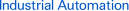# FAQ02492 of Programmable Controllers FAQ

FAQ No. FAQ02492

Question

How should I handle programs that have BCD subtraction operations that result in a negative number?

﻿

When the result of a BCD arithmetic operation is a negative number, the operation result will be output as the 10’s complement (see Additional Information, below), and the Carry Flag will be turned ON. As shown in Figure 1, immediately after executing the BCD SUBTRACTION WITHOUT CARRY (-B) instruction, the status of the Carry Flag (CY) (ON or OFF) is checked.

a. When the Carry Flag (CY) Is OFF (Operation Result Is Positive)

The execution result (D202) of the BCD SUBTRACTION WITHOUT CARRY (-B) instruction is handled as an absolute value without any further manipulation.
In this case, the sign bit (CIO 2.00) turned OFF to indicate a positive number.

b. When the Carry Flag (CY) Is OFF (Operation Result Is Negative).

Subtract the execution result (D202: 10’s compliment) of the BCD SUBTRACTION WITHOUT CARRY (-B) instruction from 0 to convert it to the actual value, and store it in D202.
In this case, the sign bit (CIO 2.00) is turned ON to indicate a negative number.

What Is a Tan's Complement?

a.Subtract a 4-digit BCD value from 9,999 or an 8-digit BCD value from 99,999,999. The ten's complement is the result with 1 added. In Figure 1, the result of 6,666 - 9,999 is -3,333, but the result that is output is the ten’s complement of 3,333 (9,999 is -3,333 + 1, or 6,667). The formula for a 4-digit BCD number is written as follows:

Complement B = 9,999 - True value A + 1 (i)

b.Formula (i) can be reversed to calculate the true value A

True value A = 10,000 - Complement B (ii)

c.The ten's complement (6,667) can be converted to the true value A (3,333) using the following formula.

10,000 - 6,667 = 3,333. The true value of complement 6,667 is 3,333. (iii)

*[-B #0 D202 D202] in Figure 1 subtracts the complement from 0000, and stores the result in D202.

(1) For 9,999 - 6,666 = 3,333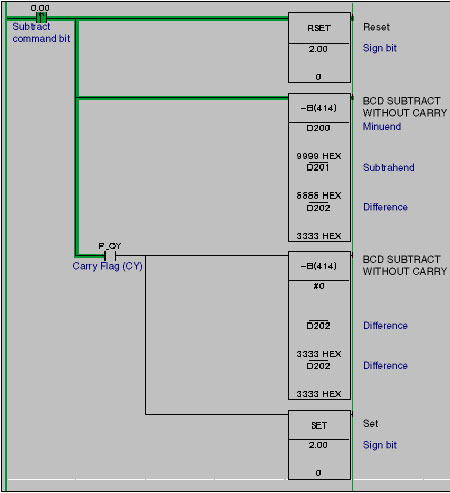Programming: CS1/CJ1-series Programmable Controllers

Figure 1

Operation

The result of the first -B instruction is, 9,999 (D200) - 6,666 (D201) = 3333 (D202) and the Carry Flag (CY) is turned OFF. For this reason, the second -B instruction will not be executed, and the operation result is 3,333 is handled as an absolute value without any further manipulation. In this case, the sign bit (CIO 2.00) is turned OFF to indicate a positive number.

BCD SUBTRACT WITHOUT CARRY (-B) Instruction

Word operands and/or constants are subtracted as 4-digit BCD values.

Carry Flag (CY)

This flag is turned ON if there is a carry in the result of the subtraction.

(2) For 6,666 - 9,999 = -3,333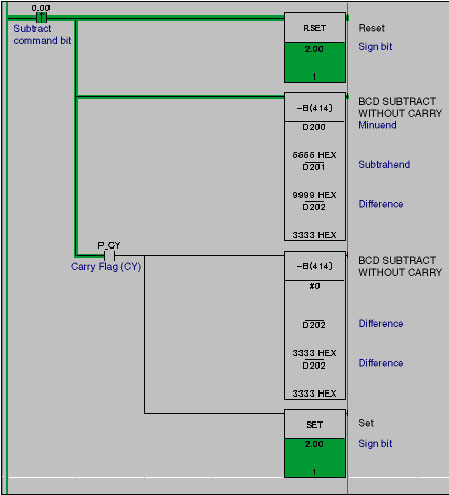Figure 2

Operation

The result of the first -B instruction execution is, 6,666 (D200) - 9,999 (D201) = 6667 (the ten's compliment of 3,333 (D202)) and the Carry Flag (CY) is turned ON. The second -B instruction will be executed, and the complement of 6,667 is converted to the true value (3,333) and stored in D202. In this case, the sign bit (CIO 2.00) is turned ON to indicate a negative number.

## Recommended Products

###CJ2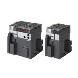Introducing the Flagship CJ2 CPU Units, with Built-in Multifunctional Ethernet Port.

###CP1E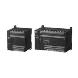Cost-effective CP1E with Enhanced Expandability for Analog and Temperature Control

###NJMotion, Logic, Safety, Vision and HMI in one